Search by Topic

Resources tagged with Spheres, cylinders & cones similar to Weird Universes:

Filter by: Content type:
Age range:
Challenge level:

There are 25 results

Broad Topics > 3D Geometry, Shape and Space > Spheres, cylinders & cones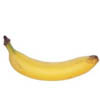Curvature of Surfaces

Age 16 to 18

How do we measure curvature? Find out about curvature on soccer and rugby balls and on surfaces of negative curvature like banana skins.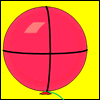Mesh

Age 16 to 18 Challenge Level:

A spherical balloon lies inside a wire frame. How much do you need to deflate it to remove it from the frame if it remains a sphere?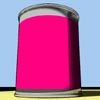Tin Tight

Age 14 to 16 Challenge Level:

What's the most efficient proportion for a 1 litre tin of paint?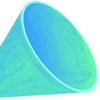Funnel

Age 14 to 16 Challenge Level:

A plastic funnel is used to pour liquids through narrow apertures. What shape funnel would use the least amount of plastic to manufacture for any specific volume ?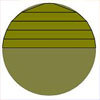Peeling the Apple or the Cone That Lost Its Head

Age 14 to 16 Challenge Level:

How much peel does an apple have?Flight Path

Age 16 to 18 Challenge Level:

Use simple trigonometry to calculate the distance along the flight path from London to Sydney.The Dodecahedron Explained

Age 16 to 18

What is the shortest distance through the middle of a dodecahedron between the centres of two opposite faces?Pythagoras on a Sphere

Age 16 to 18 Challenge Level:

Prove Pythagoras' Theorem for right-angled spherical triangles.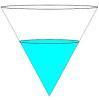Fill Me up Too

Age 14 to 16 Challenge Level:

In Fill Me Up we invited you to sketch graphs as vessels are filled with water. Can you work out the equations of the graphs?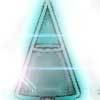Conical Bottle

Age 14 to 16 Challenge Level:

A right circular cone is filled with liquid to a depth of half its vertical height. The cone is inverted. How high up the vertical height of the cone will the liquid rise?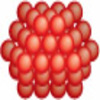Packing 3D Shapes

Age 14 to 16 Challenge Level:

What 3D shapes occur in nature. How efficiently can you pack these shapes together?Ball Packing

Age 14 to 16 Challenge Level:

If a ball is rolled into the corner of a room how far is its centre from the corner?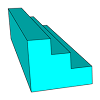Which Solid?

Age 7 to 16 Challenge Level:

This task develops spatial reasoning skills. By framing and asking questions a member of the team has to find out what mathematical object they have chosen.Air Routes

Age 16 to 18 Challenge Level:

Find the distance of the shortest air route at an altitude of 6000 metres between London and Cape Town given the latitudes and longitudes. A simple application of scalar products of vectors.Spherical Triangles on Very Big Spheres

Age 16 to 18 Challenge Level:

Shows that Pythagoras for Spherical Triangles reduces to Pythagoras's Theorem in the plane when the triangles are small relative to the radius of the sphere.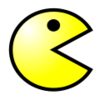Pack Man

Age 16 to 18 Challenge Level:

A look at different crystal lattice structures, and how they relate to structural properties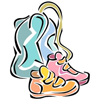Gym Bag

Age 11 to 16 Challenge Level:

Can Jo make a gym bag for her trainers from the piece of fabric she has?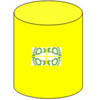Paint Rollers for Frieze Patterns.

Age 11 to 16

Proofs that there are only seven frieze patterns involve complicated group theory. The symmetries of a cylinder provide an easier approach.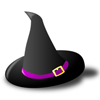Witch's Hat

Age 11 to 16 Challenge Level:

What shapes should Elly cut out to make a witch's hat? How can she make a taller hat?Immersion

Age 14 to 16 Challenge Level:

Various solids are lowered into a beaker of water. How does the water level rise in each case?2D-3D

Age 16 to 18 Challenge Level:

Two circles of equal size intersect and the centre of each circle is on the circumference of the other. What is the area of the intersection? Now imagine that the diagram represents two spheres of. . . .In a Spin

Age 14 to 16 Challenge Level:

What is the volume of the solid formed by rotating this right angled triangle about the hypotenuse?When the Angles of a Triangle Don't Add up to 180 Degrees

Age 14 to 18

This article outlines the underlying axioms of spherical geometry giving a simple proof that the sum of the angles of a triangle on the surface of a unit sphere is equal to pi plus the area of the. . . .Mouhefanggai

Age 14 to 16

Imagine two identical cylindrical pipes meeting at right angles and think about the shape of the space which belongs to both pipes. Early Chinese mathematicians call this shape the mouhefanggai.Three Balls

Age 14 to 16 Challenge Level:

A circle has centre O and angle POR = angle QOR. Construct tangents at P and Q meeting at T. Draw a circle with diameter OT. Do P and Q lie inside, or on, or outside this circle?